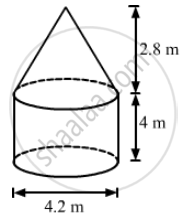Advertisement Remove all ads

# The Interior of a Building is in the Form of a Right Circular Cylinder of Diameter 4.2 M and Height 4 M Surmounted by a Cone of Same Diameter. the Height of the Cone is 2.8 M. Find the Outer - Mathematics

Sum

The interior of a building is in the form of a right circular cylinder of diameter 4.2 m and height 4 m surmounted by a cone of same diameter.
The height of the cone is 2.8 m. Find the outer surface area of the building.

Advertisement Remove all ads

#### SolutionWe have,

Radius of the cylinder = Radius of the cone = "r" = 4.2/2 = 2.1 "m",

"Height of the cylinder", "H" = 4  "m" and

Height of the  cone, h = 2.8 m

Also,

The slant height of the cone, "l" =sqrt("r"^2+"h"^2)

=sqrt(2.1^2 + 2.8^2)

=sqrt(4.41+7.84)

=sqrt(12.25)

=3.5 "m"

Now,

The outer surface area of the building = CSA 0f the cylinder + CSA of the cone

=2pi"rH" + pi"rl"

=pir(2"H"+"l")

=22/7xx2.1xx(2xx4+3.5)

=6.6xx11.5

= 75.9 m2

So, the outer surface area of the building is 75.9 m.

Is there an error in this question or solution?
Advertisement Remove all ads

#### APPEARS IN

RS Aggarwal Secondary School Class 10 Maths
Chapter 19 Volume and Surface Area of Solids
Exercise | Q 33 | Page 916
Advertisement Remove all ads
Advertisement Remove all ads
Share
Notifications

View all notifications

Forgot password?